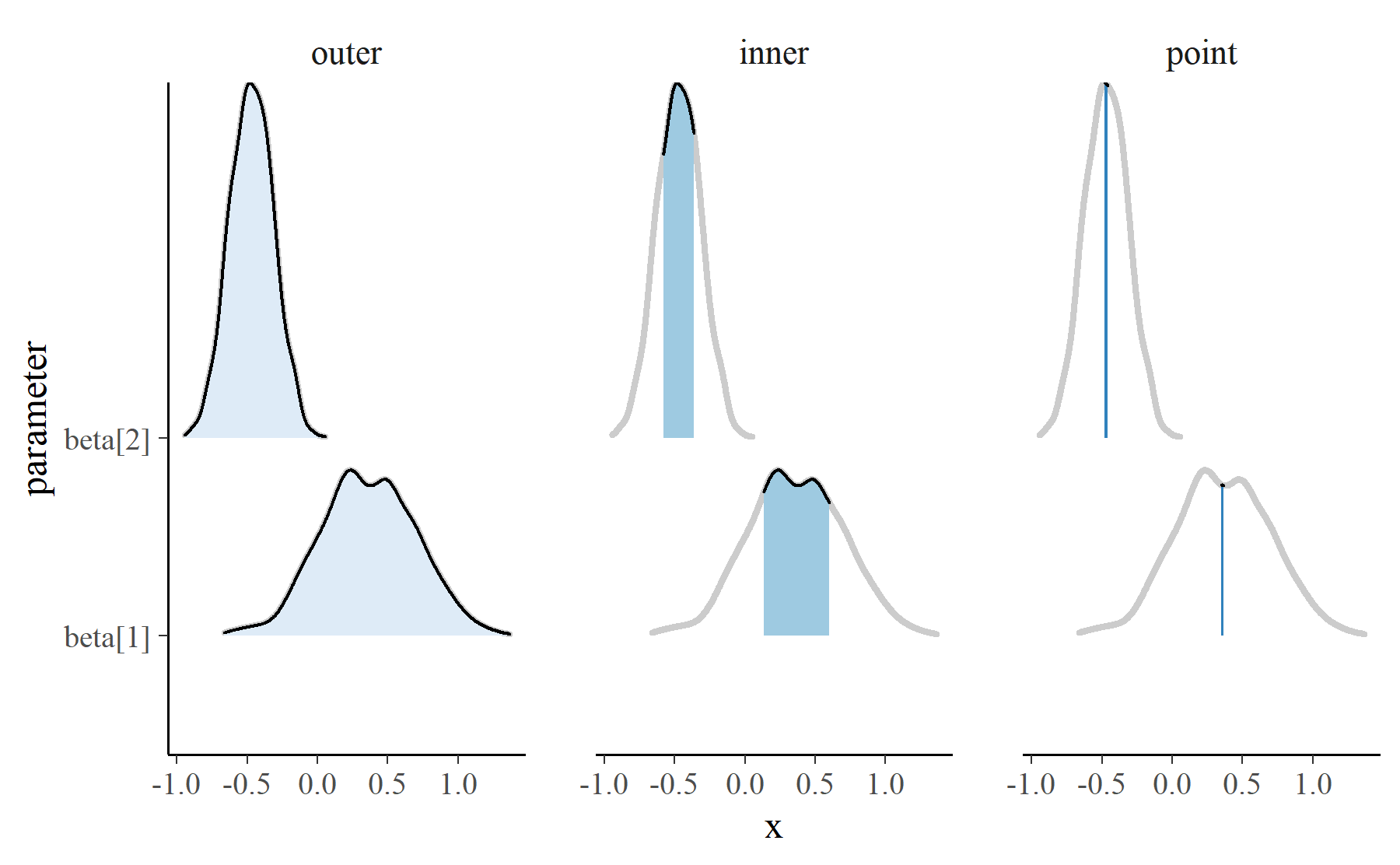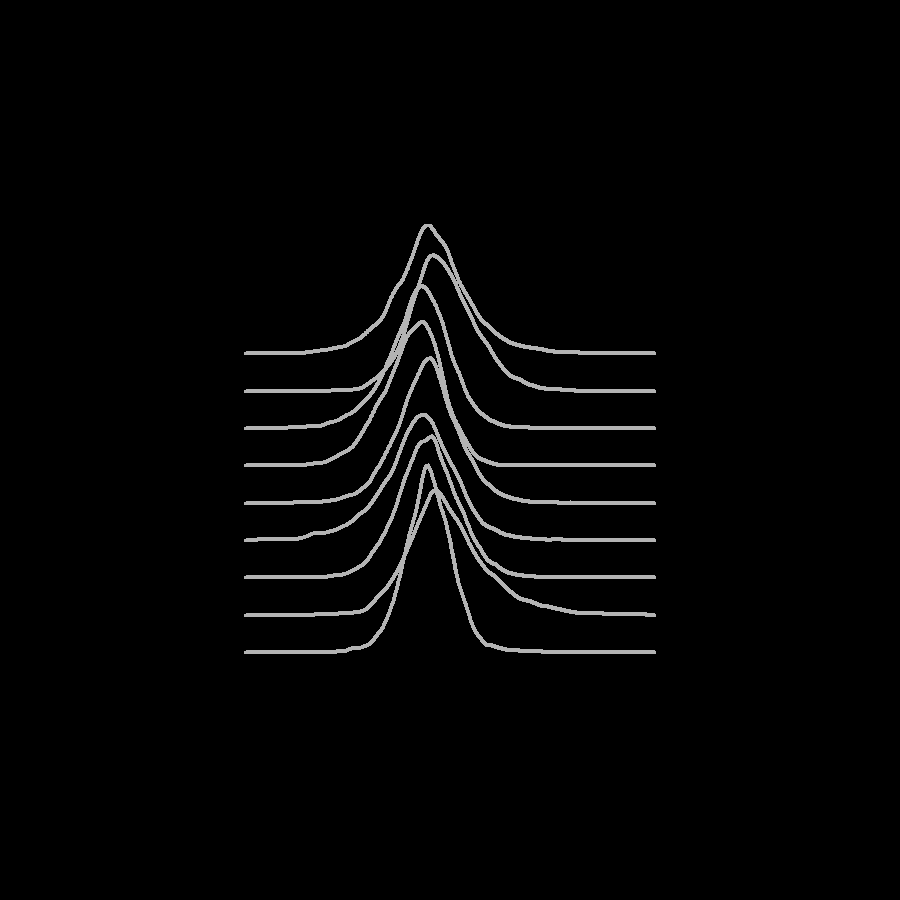# Ridgelines in bayesplot 1.5.0

April 2, 2018
By

Want to share your content on R-bloggers? click here if you have a blog, or here if you don't.

At the end of March, Jonah Gabry and I released
to the package were visualizations using ridgelines and a new plot for PIT
diagnostics from LOO validation. I don’t know what that LOO PIT thing is yet, so

## Behind the scenes of `mcmc_areas()`

`mcmc_areas()` visualizes the posterior distribution of model parameters. It
helps us see where the peak values are. By default, it will plot the complete
range of the distribution, shade the middle 50% of the distribution, and draw a
line at the median.

For this release, I rewrote the function
so that it uses the ggridges package. I had noticed that the shapes it
produced had different areas. Here’s an example of how the plots have changed.

``````library(ggplot2)

# Temporarily install the previous version of bayesplot and
# make an mcmc_areas() plot.
withr::with_temp_libpaths({
versions::install.versions("bayesplot", "1.4.0", quiet = TRUE)

example_data <- bayesplot::example_mcmc_draws()
p_a <- bayesplot::mcmc_areas(example_data, pars = c("beta", "beta")) +
ggtitle("Version 1.4.0") +
bayesplot::theme_default()
})
#> package 'bayesplot' successfully unpacked and MD5 sums checked

library(bayesplot)
#> This is bayesplot version 1.5.0
#> - Plotting theme set to bayesplot::theme_default()
#> - Online documentation at mc-stan.org/bayesplot
p_b <- mcmc_areas(example_data, c("beta", "beta")) +
ggtitle("Version 1.5.0") +
bayesplot::theme_default()

# Show the two together
cowplot::plot_grid(p_a, p_b)
``````In the plot on the left, the shapes have different areas. Eyeballing it, it
looks like `beta` would fit inside `beta`. Area is width times height.
These shapes have different widths because they cover different values—that
makes sense. Therefore, the problem is the height—namely, the fact that the
shapes have the same height. To make the areas more similar, we adjust the
heights, and that’s what’s happening in the plot on the right. The distribution
for `beta` is concentrated to a more narrow range, and that feature manifests
in that shape’s much higher peak.

### Three plots in one

This plot is built using the ggridges packages which handles drawing the shapes
at different heights. It took a bit of trickery, however, to do the annotation
for the shaded 50% interval and the median. Here is a high-level overview of
what’s happening.

`mcmc_areas_data()` tidies the MCMC samples into a dataframe suitable for
plotting. It computes the density of the parameter values, and it returns the
densities over three intervals: 1) an outer interval, 2) an inner interval to
be shaded, and 3) a narrow “point” interval.

``````library(dplyr, warn.conflicts = FALSE)

d <- mcmc_areas_data(example_data, c("beta", "beta")) %>%
mutate(
interval = factor(interval, c("outer", "inner", "point")))

d
#> # A tibble: 4,238 x 5
#>    parameter interval interval_width     x density
#>
#>  1 beta   inner             0.500 0.136   0.986
#>  2 beta   inner             0.500 0.137   0.988
#>  3 beta   inner             0.500 0.137   0.989
#>  4 beta   inner             0.500 0.138   0.990
#>  5 beta   inner             0.500 0.138   0.991
#>  6 beta   inner             0.500 0.139   0.992
#>  7 beta   inner             0.500 0.139   0.993
#>  8 beta   inner             0.500 0.139   0.994
#>  9 beta   inner             0.500 0.140   0.995
#> 10 beta   inner             0.500 0.140   0.997
#> # ... with 4,228 more rows
``````

Internally, `mcmc_areas()` uses `ggridges::geom_density_ridges()` to draw
three different ridgeline plots.

``````# Separate the full range so it can be drawn on each facet.
full_range <- d %>%
filter(interval == "outer") %>%
select(-interval)

p_base <- ggplot(d) +
aes(x = x, y = parameter, fill = interval, height = density) +
# For reference include the full-range.
# `stat = "identity"` means that ggridges should not scale the heights
ggridges::geom_density_ridges(
stat = "identity", data = full_range, color = "grey80",
size = 1, fill = NA) +
ggridges::geom_density_ridges(stat = "identity") +
scale_fill_brewer(type = "seq") +
guides(fill = FALSE)

p_base +
facet_wrap("interval")
``````These intervals are layered to achieve the desired look.There’s a bit more fussing to the actual function. For example, the width of the
intervals and whether a point is calculated are options that have to be handled.
The tips of the topmost peaks are not cut off in those plots as well. But that’s
the basic idea.

Plots with the classic, overlapping Unknown
Pleasures
look are supported
by `mcmc_areas_ridges()`. I like these plots for hierarchical models where the
parameters represent similar units. In fact, part of the reason Jonah approached
me about contributing to bayesplot was a proof-of-concept
demo
I wrote to visualize hierarchical
effects using ridgeline plots, back when they were popularly called “joyplots”.

Below is the “eight
schools”

example where there is a treatment effect for each school (thetas) and an
overall average effect (mu).

``````m <- shinystan::eight_schools@posterior_sample

mcmc_areas_ridges(m, pars = "mu", regex_pars = "theta") +
ggplot2::ggtitle("Treatment effect on eight schools (Rubin, 1981)")
``````If we really want to go for the Unknown Pleasures look, we can manually plot
the densities in a white-on-black theme. Most of the work here is padding zero
values onto the ridgelines so that they all have the same width.

``````d <- mcmc_areas_ridges_data(m, pars = "mu", regex_pars = "theta") %>%
filter(interval == "outer")

zeroes <- d %>%
# Get range of each parameter
group_by(parameter) %>%
summarise(pmin = min(x), pmax = max(x)) %>%
mutate(
min = min(pmin),
max = max(pmax),
# On each row, make a data-frame of zeroes from absolute minimum
# value to this parameter's minimum value
lower_df = purrr::map2(
min, pmin,
~ data.frame(x = seq(.x, .y, length.out = 20), density = 0)),
# Make a dataframe of zeroes from each parameter's max to the
# overall max
upper_df = purrr::map2(
pmax, max,
~ data.frame(x = seq(.x, .y, length.out = 20), density = 0)),
# Gather the zeroes together
df = purrr::map2(lower_df, upper_df, bind_rows)) %>%
select(parameter, df) %>%
tidyr::unnest(df)

ggplot(bind_rows(d, zeroes)) +
aes(x = x, y = parameter, height = density) +
ggridges::geom_density_ridges(
stat = "identity", fill = NA,
color = "grey70", scale = 5) +
theme_void() +
theme(
plot.background = element_rect(fill = "black"),
plot.margin = unit(c(.25, .25, .25, .25), units = "npc"),
axis.ticks = element_blank())
``````Finally, ridgelines appear in by-chain diagnostics using `mcmc_dens_chains()`.

``````mcmc_dens_chains(m, pars = c("theta", "theta", "theta"))
``````follows Mike DeCrescenzo’s early
example
of
using ridgelines on Bayesian models.

R-bloggers.com offers daily e-mail updates about R news and tutorials about learning R and many other topics. Click here if you're looking to post or find an R/data-science job.
Want to share your content on R-bloggers? click here if you have a blog, or here if you don't.